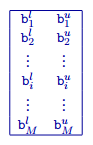# 3.3.9. Bounds File¶

This file contains an upper and lower physical property bound for each cell in the model. It is optional for the inversion program. Bounds files have the following structure:Parameter definitions:

$$b^l_{i}$$

The lower bound on the $$i^th$$ model cell.

$$b^u_{i}$$

The upper bound on the $$i^th$$ model cell.

The ordering of the cells is the same as that for model files. The total number of lines in this file should be equal to $$M$$, where $$M$$ is the total number of cells in the mesh and model. If a topography file is supplied, the bounds for cells above the surface will be ignored. It is recommended that these values be assigned a negative value (e.g. -1.0) to avoid confusion.